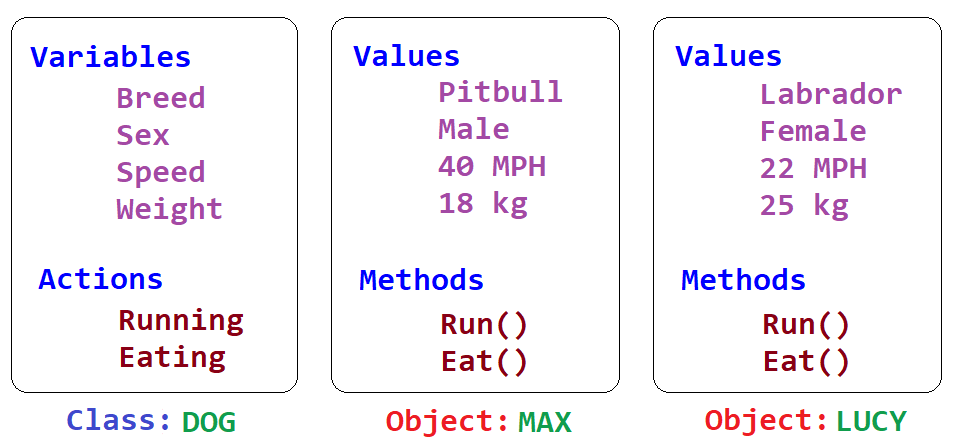# Classes and Objects in Python Programming

###### OOPs in Python

In this lesson, we will understand what is Classes and Objects in Python Programming and how to create them along with some examples.

## What is Class and Object in Python Programming?

A Class is a user-defined data type that contains data (variables) and methods (functions) together. An Object is an instance or part of a class.

Let's understand Class and Object using a real life example.In the above image, we can see that DOG is a class, or we can say it's a group. At the same time, MAX and LUCY are objects of the class DOG.

An object contains the same variables as its class but may store different values. For example, MAX is an object of the class DOG having variables and its values as Breed: Pitbull, Sex: Male, Running Speed: 40 MPH, and Weight: 18 Kg. LUCY is another object of the class DOG having variables and its values as Breed: Labrador, Sex: Female, Running Speed: 22 MPH, and Weight: 25 Kg.

We can treat Actions as the processing of data stored in the variables of an object. For example, using the action Running, we can measure the speed of a dog. Similarly, using the action of Eating, a dog's weight can be measured. In programming, we can code these actions as a function inside a class.

When we write a function inside a class, it is called Method.## Creating a Class in Python

We create a class by using the keyword class and then writing the class name with a colon : at the end.

### Syntax for creating a class in python

``class class_name:``

#### Example

``class Record:``

Here Record is the name of a class. Generally, a class name should start with a capital letter, as discussed in the lesson Python Naming Conventions.

## Creating an Object of a Class in Python

We create an object by writing the object name with an equal sign = and then writing the class name with a pair of parenthesis () at the end.

### Syntax for creating an object of a class in python

``object_name = class_name()``

#### Example

``x = Record()``

Here x is the name of an object of the class Record(). We can not create an object if the class is empty.

We know how to create a class and its object in python, but a class can not be kept empty, so we need to declare some variables and methods in it to make it helpful.

In our following lessons, we will learn how to create different types of variables and methods in a class to store and process data.